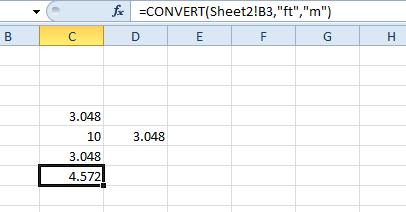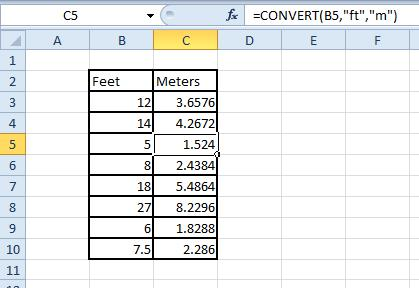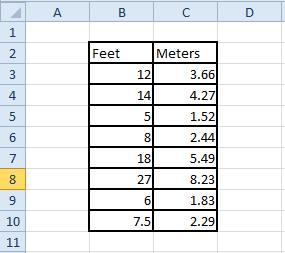# How To Convert Feet to Meters in Excel

You can convert a range of values to meters with a spreadsheet application. Excel is one of the best software packages with which to convert a variety of units to other alternatives. This is how you can set up a foot to meters conversion spreadsheet in Excel.### Convert Feet to Meters Without a Function

One foot is equal to 0.3048 meters. To convert feet to meters with a calculator, you multiply feet by 0.3048. There are also 3.28 feet in a meter, so another way to convert foot to meter is to divide the number of feet by 3.28. So you can convert feet to meters with either of the two formulas below:

Ft x 0.3048 = m

Ft / 3.28 = m

You can add either of those formulas to an Excel spreadsheet cell. As an example, open a blank spreadsheet and select cell C4. Then click inside the fx bar, enter ‘=10*0.3048’ and press the Return key. The spreadsheet will return the value of 3.048 meters in C4 as shown below.You can also add cell references to that formula to convert values entered in a spreadsheet to meters. Select cell C5 and input ’10’ as the feet value. Then select cell D5 and enter the formula ‘=C5*0.3048’ in the fx bar. D5 will then return the value of 3.048 meters.

### Convert Feet to Meters With Convert

CONVERT is the best Excel function for unit conversion. This function enables Excel users to convert a variety of distance, volume, time, energy, volume and area units. As such, you can also convert feet values to meters with the function. The function’s syntax is: CONVERT(number, from_unit, to_unit).

To add this function to your spreadsheet, select cell C6. Press the fx button to open the Insert Function window. Select All from the Or select a category drop-down menu. Select the CONVERT function and click OK to open the window in the snapshot below.First, press the Number cell reference button and select C5, where you entered 10 as the feet value. Then input “ft” in the From_unit field. Enter “m” in the To_unit box. Press the OK button to add this function to cell C6. That will now include the value 3.048 meters, which amounts to 10 feet.You can also add the function to spreadsheets without including any cell references in it. To do that, you’ll need to replace the function’s cell reference with a foot value. For example, you could select a cell and enter ‘=CONVERT(10, “ft”, “m”)’ in the fx bar. That will also convert 10 feet to meters.

You can also convert values that aren’t included in the same Excel sheet as the CONVERT function. For example, click the Sheet2 tab at the bottom of the spreadsheet window; and input the feet value ’15’ in cell B3. Then return to Sheet1, select cell C7 and click in the fx bar. Input ‘=CONVERT(sheet2!B3,“ft”,“m”)’ in the fx bar and press Enter. C7 in Sheet1 will convert the value of cell B3 in Sheet2 to meters and return 4.572.### Set up a Conversion Spreadsheet

Now you can set up a foot to meters conversion spreadsheet table with the CONVERT function. Open a blank Excel spreadsheet, and input ‘Feet’ in cell B2. Enter ‘Meters’ in cell C2 as a table heading for the column that will include the CONVERT function.

Next, enter all the feet values to convert to meters in column B. Select cell C3 and enter ‘=CONVERT(B3,”ft”,”m”)’ in the function bar. That will convert the B3 value to meters only, but you can copy the function to all the column cells below it. Left-click the bottom corner of cell C3 and drag the box over the cells in column C that you need to copy the function to. Then your table should look something like the one below.To finish the table, add a little formatting to it. Select all the table’s cells by pressing the left mouse button and dragging the cursor over them. Then you should right-click to open the context menu, select Format Cells and click Border. Select a line outline to add to the table, and press the Outline and Inside preset buttons. Press OK to apply the formatting to the table as below.You can also round the numbers down in the Meters column to a couple of decimal places. To do that, select the cells and open the Format Cells window again. Click the Number tab, select Number and then enter ‘2’ in the Decimal places text box. Press OK to round the values down to a couple of decimal places as below.Now you have a spreadsheet that converts feet to meters! You can always expand the table to add more feet values when required. Check out this Tech Junkie guide for details on how to convert feet to inches with the CONVERT function and Kutools add-on in Excel.

## One thought on “How To Convert Feet to Meters in Excel”dick says:
gay

Disclaimer: Some pages on this site may include an affiliate link. This does not effect our editorial in any way.

Todays Highlights